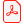# Conference article

## Modelling GME and PLS Estimation Methods for Evaluating the Job Satisfaction in the Public Sector

Enrico Ciavolino
Researcher of Statistics, University of Salento, Department of Philosophy & Social Science, Italy

Download articleLinköping Electronic Conference Proceedings 26:43, p.

Published: 2008-02-15

ISBN:

ISSN: 1650-3686 (print), 1650-3740 (online)

### Abstract

Golan et al. (1996) proposed an alternative method for the parameters estimation of the regression models; in case of ill-posed problems; as an extension of the entropy measure; introduced by Shannon and as a generalization of the Maximum Entropy Principle (MEP) developed by Jaynes (1957; 1968).

The job satisfaction model is considered as motivating example; for developing a performance comparative study between the GME and PLS regression methods. Both GME and PLS methods are implemented on the job satisfaction model; where several level of correlation are generated among the predictors. For each level of correlation; regression coefficients and diagnostic values are calculated; for showing the performance of both methods in case of ill-posed problems.

The paper is divided in two main sections: The first part consider the introduction of the two estimation methods; in way to give a general overview of both techniques and also the main characteristics.

The second part gives a brief introduction to the job satisfaction model and then starts with the simulation study; comparing both estimation results; giving a discussion in case of multicollinearity problem.

### Keywords

Generalized Maximum Entropy; Partial Least Squares; Job Satisfaction; Multicollinearity; Bootstrap

### References

Al-Nasser A. D. (2003). Customer Satisfaction Measurement Models: Generalized Maximum Entropy Approach. Pak Journal of Statistics; 19(2); 213–226;

Belsley; DA; E Kuh & RE Welsch (1980). Regression Diagnostics; Identifying Influential Data and Sources of Collinearity; Wiley; New York;

Ciavolino E. (2007). The Entropy Theory for evaluating the Job Satisfaction; GFKL 2007; Freiburg; march 2007;

Ciavolino E.; Al Nasser A.D.; D’Ambra A. (2006). The Generalized Maximum Entropy Estimation method for the Structural Equation Models; GFKL 2006; Berlino; marzo 2006;

Golan; A; G George & D Miller (1996). Maximum Entropy Econometrics; Wiley; London;

Höskuldsson A (1988); PLS Regression Methods; Journal of Chemometrics; 2; 211- 228;

Jaynes E.T. (1957). Information Theory and Statistical Mechanics; The Physical Review 106 (4); 620-630; May 15; 1957;

Jaynes; E. T. (1968). Prior Probabilities; IEEE Transactions On Systems Science and Cybernetics; vol. sec-4; no. 3; 227-241;

Paris; Q. (2001): Multicollinearity and maximum entropy estimators. Economics Bulletin; 3(11); 1–9;

Shannon C. E. (1948). A mathematical Theory of Communications; Bell System Technical Journal; 27; 379–423;

Wold S.; Sjostrom M.; Eriksson L.; (2001); PLS-regression: a basic tool of chemometrics; Chemometrics and Intelligent Laboratory Systems; vol. 58; 109- 130;